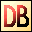DelphiBasics
 RectFunction Create a TRect value from 2 points or 4 coordinates Classes unit
 1 function Rect(Left, Top, Right, Bottom Integer):TRect; 2 function Rect (TopLeft, BottomRight TPoint:TRect;
Description
The Rect function creates a TRect (rectangle) value from either 4 coordinates or 2 points.

When creating from two points TopLeft and BottomRight, you can pass two TPoint values, or use the Point function to generate them.
Notes
Important

There are two Rect functions in the Classes and Types units. Only the former supports the second syntax.

If using both of these units in your code, and you specify Types after Classes you must prefix Rect with Classes to use this second syntax.
Related commands
 Bounds Create a TRect value from top left and size values Point Generates a TPoint value from X and Y values PointsEqual Compares two TPoint values for equality PtInRect Tests to see if a point lies within a rectangle TPoint Holds X and Y integer values TRect Holds rectangle coordinate valuesDownload this web site as a Windows program.

 Example code : Create two rectangles using both syntaxes var   rectangle1, rectangle2 : TRect; begin   // Set up the first rectangle using syntax 1   rectangle1 := Rect(20, 40, 60, 80);   // Set up the second rectangle using the Rect function   rectangle2 := Classes.Rect(Point(20, 40), Point(60, 80));   // Display the top left and bottom right coords of each rectangle   ShowMessageFmt('Rectangle 1 coords = %d,%d,%d,%d',                  [rectangle1.Left,                   rectangle1.Top,                   rectangle1.Right,                   rectangle1.Bottom]);   ShowMessageFmt('Rectangle 2 coords = %d,%d,%d,%d',                  [rectangle2.Left,                   rectangle2.Top,                   rectangle2.Right,                   rectangle2.Bottom]); end; Show full unit code Rectangle 1 coords = 20,40,60,80   Rectangle 2 coords = 20,40,60,80